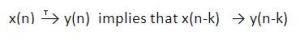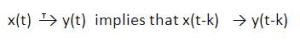# Time Variant or Time Invariant Systems

## Time Variant or Time Invariant Systems

### Definition:

A system is said to be Time Invariant if its input output characteristics do not change with time. Otherwise it is said to be Time Variant system.

### Explanation:

As already mentioned time invariant systems are those systems whose input output characteristics do not change with time shifting. Let us consider x(n) be the input to the system which produces output y(n) as shown in figure below.

Now delay input by k samples, it means our new input will become x(n-k). Now apply this delayed input x(n-k) to the same system as shown in figure below.

Now if the output of this system also delayed by k samples (i.e. if output is equal to y(n-k)) then this system is said to be Time invariant (or shift invariant) system.

If we observe carefully, x(n) is the initial input to the system which gives output y(n), if we delayed input by k samples output is also delayed by same (k) samples. Thus we can say that input output characteristics of the system do not change with time. Hence it is Time invariant system.

### Theorem:

A system is Time Invariant if and only ifSimilarly a continuous time system is Time Invariant if and only ifNow let us discuss about How to determine that the given system is Time invariant or not?

To determine whether the given system is Time Invariant or Time Variant, we have to follow the following steps:

Step 1: Delay the input x(n) by k samples i.e. x(n-k). Denote the corresponding output by y(n,k).

That means x(n-k)  ? y(n,k)

Step 2: In the given equation of system y(n) replace ‘n’ by ‘n-k’ throughout. Thus the output is y(n-k).

Step 3: If y(n,k) = y(n-k) then the system is time invariant (TIV) and if y(n,k) ? y(n-k) then system is time variant (TV).

Same steps are applicable for the continuous time systems.

### Solved Problems:

1)      Determine whether the following system is time invariant or not.

y(n) = x(n) – x(n-2)

Solution:

Step 1: Delay the input by ‘k’ samples and denote the output by y(n,k)

Therefore y(n,k) = x(n-k) – x(n-2-k)

Step 2: Replace ‘n’ by ‘n-k’ throughout the given equation.

Therefore y(n-k) = x(n-k) – x(n-k-2)

Step 3: Compare above two equations. Here y(n,k) = y(n-k). Thus the system is Time Invariant.

2)      Determine whether the following systems are time invariant or not?

y(n) = x(n) + n x(n-2)

Solution:

Step 1: Delay the input by ‘k’ samples and denote the output by y(n,k)

Therefore y(n,k) = x(n-k) + n x(n-2)

Step 2: Replace ‘n’ by ‘n-k’ throughout the given equation.

Therefore y(n-k) = x(n-k) + (n-k) x(n-k-2)

Step 3: Compare above two equations. Here y(n,k) ? y(n-k). Thus the system is Time Variant.

### You May Also Like:

1.In 2 question why nx(n-2) isn't replaced by (n-2)x(n-2-k) in case of of finding y(n,k) as it's also the input?

2.By using these steps, y(n)=x(2n) will become time invarient but we now that it is varient becouse shift become double im output.. .

3.How to delay y(n)=x(2n) by k ????

4.Anirban ChakrabortyNovember 27, 2017 at 1:24 PM

Y(n,k) = x(2n-k)
Y(n-k) = x(2{n-k})
= x(2n-2k)

So time variant

5.sir is it possible to check the system is time variant or invariant using c program? If yes then how to write the code.

6.Electrical TechnicianNovember 13, 2018 at 6:49 AM

Yes, why?!! .. I mean there must be expression, we ask the writer

7.x^2(n) time variant or invariant ?

8.time invariant

9.Time Invariant